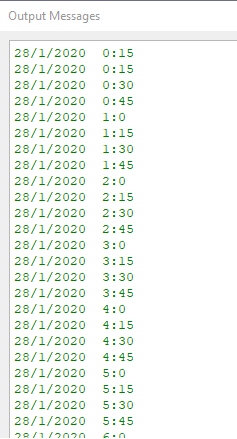# How to execute a python script every step of an animation?

Hi,

I need to execute some python code (using the TimeKeeper.Time) every timestep of the animation
After some research I have tried(unsucessfully):

``````rv = GetActiveView()
``````

Any tips on how can I do this?

You can add a Python animation track from the Animation View.

1 Like

Thank you, Utkarsh but it is not exactly what I am looking for because the variables of the code are reset every timestep.

The goal is to show the date of the simulation in the screen, using a start date and updating it every timestep, since the animation track run every timestep I’ve removed the part with TimeKeeper.Time

Python animation try code:

``````day = 28
month = 1
year = 2020
hour = 0
minute = 0
unitOfTime = 15

def start_cue(self):
global day
global month
global year
global hour
global minute
global unitOfTime
minute += unitOfTime
if(minute == 60 ):
hour+=1
minute = 0
if(hour == 24):
day+=1
hour = 0
if(day == 32):
month+=1
day = 1
if(month == 13):
year+=1
month = 1
return str(day) + '/' + str(month) + '/' + str(year) + '  ' + str(hour) + ':' + str(minute)

def tick(self): pass
def end_cue(self): pass
``````

Code before the changes:

``````from paraview.simple import *
from paraview.vtk import vtkCommand

rv = GetActiveView()
tk = GetTimeKeeper()
dia = 28
mes = 1
ano = 2020
hora = 0
minuto = 0
timeStep = tk.Time
nextTimeStep = timeStep+1

global dia
global mes
global ano
global hora
global minuto
global timestep
global nextTimeStep
if(timeStep >= nextTimeStep):
nextTimeStep+=1
if(minuto == 60 ):
hora+=1
minuto = 0
if(hora == 24):
dia+=1
hora = 0
if(dia == 32):
mes+=1
dia = 1
if(mes == 13):
ano+=1
mes = 1
return str(dia) + '/' + str(mes) + '/' + str(ano) + '  ' + str(hora) + ':' + str(minuto)

def callback(caller, event):
timeStep = tk.Time

text1 = Text()
text1Display = Show(text1, rv, 'TextSourceRepresentation')
``````

Here’s a little bit of a hack to preserve some state.

``````global foo

def start_cue(self):
global foo
foo = 12

def tick(self):
global foo
foo  += 1
print("foo=", foo)

def end_cue(self): pass
``````

I’ve reported an issue here. That should fix this idiosyncrasy.

Worked flawlessly! Thank you!The thing is, the goal is to show these outputs in a Text on the RendeView something like

``````text1 = Text()
text1Display = Show(text1, rv, 'TextSourceRepresentation')
text1.Text = outputOfTheTimeStep
``````

Does the Python Animation Track gives me this possibility in any manner?

something like this could work

``````global foo

def start_cue(self): pass
def end_cue(self): pass

def tick(self):
global foo
try:
textSource = foo
except NameError:
from paraview.simple import Text, Show
textSource = Text()
foo = textSource
Show(textSource)
finally:
textSource.Text = str(self.GetAnimationTime())
``````
1 Like